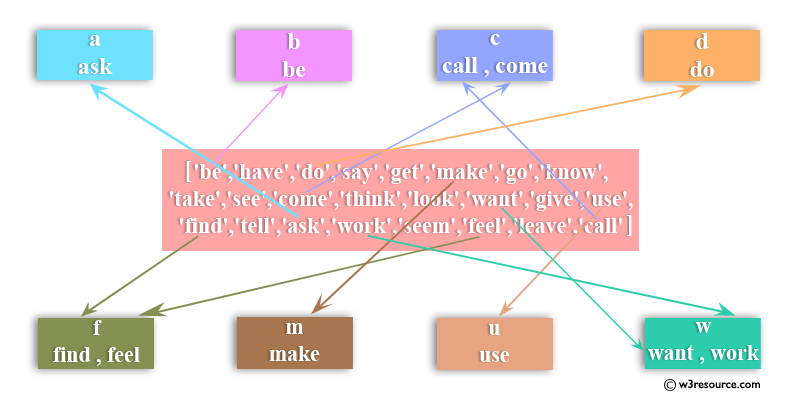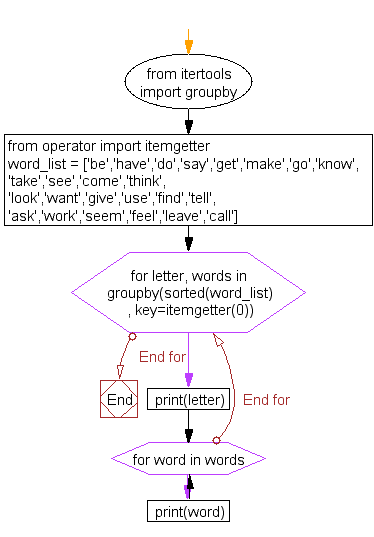﻿ Python: Split a list based on first character of word - w3resource# Python: Split a list based on first character of word

## Python List: Exercise - 40 with Solution

Write a Python program to split a list based on first character of word.

Sample Input:

```word_list = ['be','have','do','say','get','make','go','know','take','see','come','think',
```Sample Solution:-

Python Code:

``````from itertools import groupby
from operator import itemgetter

word_list = ['be','have','do','say','get','make','go','know','take','see','come','think',

for letter, words in groupby(sorted(word_list), key=itemgetter(0)):
print(letter)
for word in words:
print(word)
```
```

Sample Output:

```a
b
be
c
call
come
d
do
-----
w
want
work
```

Flowchart:## Visualize Python code execution:

The following tool visualize what the computer is doing step-by-step as it executes the said program:

Python Code Editor:

Have another way to solve this solution? Contribute your code (and comments) through Disqus.

What is the difficulty level of this exercise?

Test your Python skills with w3resource's quiz

﻿

## Python: Tips of the Day

Floor Division:

When we speak of division we normally mean (/) float division operator, this will give a precise result in float format with decimals.

For a rounded integer result there is (//) floor division operator in Python. Floor division will only give integer results that are round numbers.

```print(1000 // 300)
print(1000 / 300)```

Output:

```3
3.3333333333333335```